# Electrical Engineering test questions for exams and entrance - 9

## Electrical Engineering test questions for exams and entrance - 9

(1) Consider the figure shown below. The steady state error of a closed loop control system when subjected to a unit step input is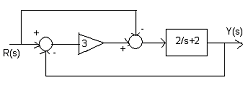(A) 0
(B) 1
(C) -1
(D) -1.5

(2) The sampling wattmeter that computes power from simultaneously sampled values of voltage and current is used to measure the average power of the load. As shown in the figure, 10 V is

the peak-to-peak voltage of the square wave and 5A is the peak-to-peak current of the triangular wave. The period is of 20ms. What will be the reading?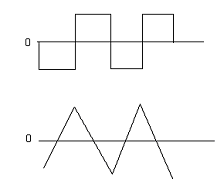(A) 1 W
(B) 0 W
(C) 100 W
(D) 50 W

For Questions 3, 4 and 5 refer to the data given below:

A double layer winding is housed in a 48 slots of a 4-pole, 50Hz, synchronous generator. 10 turns is present in each coil and is short pitched by an angle to 36° electrical. The

fundamental flux per pole is 0.025 Wb.

(3) For a 3-phase star connection, the approximate value of line-to-line induced emf is

(A) 440 V
(B) 500 V
(C) 800 V
(D) 1400 V

(4) For a 2-phase connection, the approximate value of line-to-line induced emf is

(A) 950 V
(B) 1000 V
(C) 1143 V
(D) 1200 V

(5) For a 3-phase star connection, the 5th harmonic component of phase emf is

(A) 220 V
(B) 0 V
(C) 1 V
(D) None of the above

(6) In 8085 microprocessor, the assembly language instruction that stores the content of registers H and L into the respective memory location 2050? and
2051? is

(A) SPHL 2051?
(B) SHLD 2050?
(C) STAX 2050?
(D) SPHL 2050?

(7) Self reactance and mutual reactance of a transposed overhead transmission line are 0.4 Ω/km and 0.1Ω/km respectively. The respective values of positive sequence reactance

and zero sequence reactance (in Ω/km) are

(A) 0.3 and 0.6
(B) 0.1 and 0.3
(C) 0.5 and 0.2
(D) 0.9 and 0.6

(8) For the figure shown below, Ri = 1MΩ, Ro = 10Ω, A = 106 V/V. If Vi = 1μV, then output voltage, input voltage and output impedance respectively are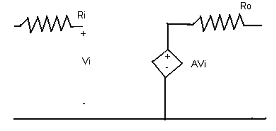(A) 1 V, 0, ∞
(B) 1 V, 0, 10 Ω
(C) 1 V, ∞, 10 Ω
(D) 1 V, ∞, 10 Ω

ANSWER: 1 V, ∞, 10 Ω

(9) The figure shows the feedback system where K > 0. What should be the range of K for the system to be stable?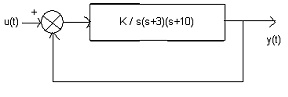(A) 0(B) 0(C) 0(D) K>390

(10) The resolution of a digital-to-analog converter is close to 14mV. The full-scale output voltage of the converter is 3.5 V. What will be its bit size?

(A) 16
(B) 24
(C) 4
(D) 8

For Questions 11 and 12 refer to the data given below.

Consider a synchronous motor whose synchronous reactance is 1.0 pu and negligible resistance. It is connected to an infinite bus at 1.0pu voltage and draws 0.6pu current at unity power

factor.

(11) The respective value of excitation voltage and load angle will be

(B) 1.17pu and 30.96° lag
(D) 0.54pu and 35.12° lag

(12) Keep excitation voltage same. The load on the motor is increased such that the motor current increases by 20%. The operating power factor will be

(A) 0.848 Lagging
(C) 0.974 lagging

(13) Consider an industrial sub-station with a 4 MW load. To maintain the load power factor at 0.97 lagging, a capacitor of 2 MVAR is installed in the sub-station. What will happen if the

capacitor goes out of service?

(A) The load power factor becomes 1.00
(B) The load power factor becomes 0.90 lag
(C) The load power factor becomes 0.80 lag
(D) The load power factor becomes 0.80

For questions 14, 15 and 16 refer to the data given below:

The starting current of a 3-phase squirrel cage induction motor is seven times the full load current and full load slip is of 5%.

(14) What should be the autotransformer ratio if an autotransformer is used for reduced voltage starting to provide 1.5 per unit starting torque?

(A) 75.50%
(B) 78.25%
(C) 80.65%
(D) 50.23%

(15) What will be the per unit starting torque if a star-delta starter is used to start this induction motor?

(A) 1.239
(B) 0.576
(C) 0.816
(D) 1.538

(16) What should be the per unit starting current if a starting torque of 0.5 per unit is required?

(A) 2.35
(B) 4.96
(C) 3.72
(D) 3.16

(17) Consider the digital circuit shown below. As long as x1 = x2 = 1, the output Q remains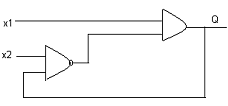(A) Unstable
(B) At its initial value
(C) At 1
(D) At 0

(18) A star connected 12√3 kVA, 0.8 pf (lag) load is power supplied by a 400V, 50 Hz 3-phase balanced source. To bring the

pf to unity, the rating of the delta connected capacitive reactive power bank is

(A) 24.32 kVAR
(B) 12.47 kVAR
(C) 15.26 kVAR
(D) 30.30 kVAR

(19) Consider a single-phase fully controlled bridge converter. It supplies a load drawing constant and ripple free load current. What will be the input power factor if the triggering

angle is 30°?

(A) 0.56
(B) 0.93
(C) 0.78
(D) 0.88

(20) Figure shows the root locus plot of a third order system (Location of poles are not given). The open loop transfer function will be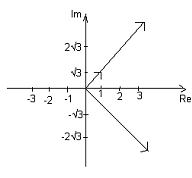(A) K / s² (s + 1)
(B) K / s (s² + 1)
(C) K / (s² + 1)
(D) K / s³

(21) Which one of the following statement is true? In a transformer, zero voltage regulation at full load is

(A) Not at all possible
(B) Possible at unity pf load
(C) Possible at lagging pf load

(22) The step angle of a 3-phase, 3-stack variable reluctance step motor that has 20 poles on each rotor and stator stack is

(A) 6°
(B) 3°
(C) 18°
(D) 10°

(23) Consider an 8085 microprocessor based system. The contents of memory location whose address is available in (D,E) register pair is desired to be incremented and the result is to be

stored in the same location. The sequence of instructions to be used is

(A) XCHG
INX H
(B) XCHG
INR M
(C) INR M
XCHG
(D) INX D
XCHG

INR M

For Questions 24 and 25 refer to the data given below:

The synchronous reactance of a 1000kVA, 6.6 kV, 3-phase star connected cylindrical pole synchronous generator is 20Ω. Assume operation at full load with unity power factor and armature

resistance is negligible.

(24) Line-to-line induced emf is close to

(A) 7.2kV
(B) 6.4kV
(C) 10.5kV
(D) 1.6kV

(25) The power (or torque) angle is close to

(A) 18.2°
(B) 24.6°
(C) 36.0°
(D) 12.2°